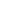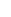# How to bend an arc with a WC67K-200T3200 Hydraulic Press Brake machine

Views: 3     Author: DURMAPRESS     Publish Time: 2022-07-29      Origin: DURMAPRESSHow to bend an arc with a WC67K-200T3200 Hydraulic Press Brake machine

It can be bent slightly, so that the arc can be folded, the detailed steps are as follows:

1. As shown in the red circle, put the place where you want to bend the arc under the bending machine.

2. Press the switch and press down the bending machine.3. As shown in the red circle in the figure, after the bending machine presses the iron sheet, the hand only needs a small amount of lifting, so that it will not form too large an Angle. 4, then lift the bending machine, send the iron sheet forward, repeat the above three steps, according to the size of the arc you want to repeat three times, or more than three times.

5, here, we repeat three times, fold a semicircle arc. 6, as shown in the picture, so that you can fold an arc, visible effect is good.Each feeding algorithm: plate thickness 2mm bending Angle 120 degrees bending external radius 30 neutral layer radius 29, arc bending we calculate to expand the arc length of the neutral layer. Therefore, the number of bending knives and bending Angle of each knife are also calculated according to the neutral arc length of the neutral layer and the distance between the neutral layer and the edge is half of the plate thickness. How many cuts are required to bend the arc in the figure above, and what is the bending Angle of each cut? If we cut 2mm each way.Number of curved blades = arc length /2mm=30.37/2=15 Number of curved blades 15 bending Angle =180- {(2/ arc length)X (180- bending Angle)}=180-{(2/30.37)X60}=176 Bending Angle per knife 176 degrees if we can not measure the arc length when bending operation, we can also calculate the bending Angle according to the following formula =180-{(2/3.14x neutral layer radius) X180}=180-{(2/3.1) If you want to make every 3mm, change the 2 to 3 in the above formula, which is of course the theoretical formula. In actual processing, the results calculated by the above formula are adjusted.0086 555 8327689+86 18325572889info@durmapress.com